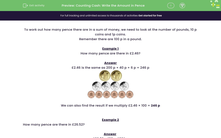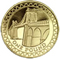# Convert Pounds and Pence into Pence

In this worksheet, students will recognise and state how many pence in total there are in a given amount of pounds and pence.Key stage:  KS 2

Curriculum topic:   Measurement

Curriculum subtopic:   Use Different Measures

Popular topics:   Money worksheets

Difficulty level:#### Worksheet Overview

In this activity, we will be working out how many pence there are in a sum of money by looking at the number of pounds, 10p coins and 1p coins.

Remember that there are 100p in a pound.

Example 1

How many pence are there in £2.46?

£2.46 is the same as 200p + 40p + 6p = 246pWe can also find the result if we multiply £2.46 × 100 = 246p

Or simply remove the decimal point!

Example 2

How many pence are there in £26.52?

£26.52 × 100 = 2652p

Top tip: The decimal point separates the pounds and the pence, so if we remove it, we end up with just the pence!(In the following questions, leave out the p in the answers)

### What is EdPlace?

We're your National Curriculum aligned online education content provider helping each child succeed in English, maths and science from year 1 to GCSE. With an EdPlace account you’ll be able to track and measure progress, helping each child achieve their best. We build confidence and attainment by personalising each child’s learning at a level that suits them.

Get started